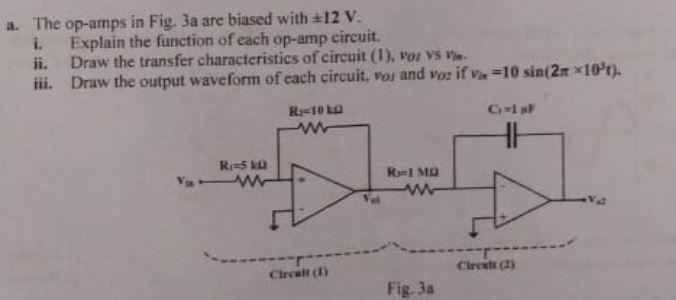# a. The op-amps in Fig. Ja are biased with #12 V. 1. Explain the function of each op-amp circuit. ii. Draw the transfer charac The op-amps in fig.3a are biased with +_12V i.Explain The Function of each op-amp circuit. ii. Draw the transfer characteristics of Circuit (1),Vo1 Vs Vin iii. Draw The output waveform of each circuit, Vo1 and Vo2 if Vin=10sin(2pi*10^3t)

Question-AnswerCategory: Electrical Engineeringa. The op-amps in Fig. Ja are biased with #12 V. 1. Explain the function of each op-amp circuit. ii. Draw the transfer charac The op-amps in fig.3a are biased with +_12V i.Explain The Function of each op-amp circuit. ii. Draw the transfer characteristics of Circuit (1),Vo1 Vs Vin iii. Draw The output waveform of each circuit, Vo1 and Vo2 if Vin=10sin(2pi*10^3t)The op-amps in fig.3a are biased with +_12V
i.Explain The Function of each op-amp circuit.
ii. Draw the transfer characteristics of Circuit (1),Vo1 Vs Vin
iii. Draw The output waveform of each circuit, Vo1 and Vo2 if Vin=10sin(2pi*10^3t)